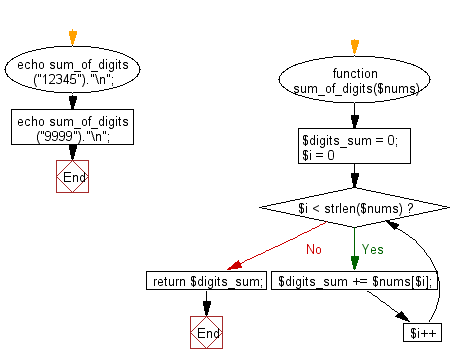﻿ PHP Exercise: Compute the sum of the digits of a number - w3resource

# PHP Exercises: Compute the sum of the digits of a number

## PHP: Exercise-45 with Solution

Write a PHP program to compute the sum of the digits of a number.

Sample Solution: -

PHP Code:

``````<?php
function sum_of_digits(\$nums) {
\$digits_sum = 0;
for (\$i = 0; \$i < strlen(\$nums); \$i++) {
\$digits_sum += \$nums[\$i];
}
return \$digits_sum;
}
echo sum_of_digits("12345")."\n";
echo sum_of_digits("9999")."\n";
?>
```
```

Sample Output:

```15
36
```

Flowchart:PHP Code Editor:

Have another way to solve this solution? Contribute your code (and comments) through Disqus.

What is the difficulty level of this exercise?

Test your Programming skills with w3resource's quiz.

﻿

## PHP: Tips of the Day

Returns all elements in an array except for the first one

Example:

```<?php
function tips_tail(\$items)
{
return count(\$items) > 1 ? array_slice(\$items, 1) : \$items;
}

print_r(tips_tail([1, 5, 7]));
?>
```

Output:

```Array
(
 => 5
 => 7
)
```